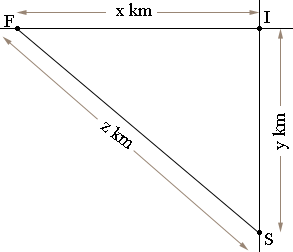SEARCH HOMEMath Central Quandaries & QueriesQuestion from Michael, a student: Hi there, I'm in grade 12 and I'd like some help in approaching this problem. Two cars approach a right-angled intersection, one traveling south a 40km/h and the other west at 70km/h. When the faster car is 4km from the intersection and the other case if 3km from the intersection, how fast is the distance between the car cars changing? Many thanks in advance! MichaelHi Michael,

In my diagram I is the intersection, F is the position of the fast car and S is the position of the slow car at some time $t$ hours. The distances from the cars to the intersection and the distance between the cars are changing so $x, y$ and $z$ are functions of time, that is $x = x(t), y = y(t)$ and $z = z(t).$Since $IFS$ is a right triangle Pythagoras theorem gives us that

$x(t)^2 + y(t)^2 = z(t)^2$

Differentiate both sides of the equation with respect to $t$ to obtain an equation containing $x(t), x'(t), y(t), y'(t), z(t)$ and $z'(t).$ You know that $x'(t) = 70$ km/hr and $y'(t) = 40$ km/hr. Also at some particular time $t_0$ you know that $x(t_0) = 4$ km and $y(t_0) = 3$ km. What are $z(t_0)$ and $z'(t_0)?$

PennyMath Central is supported by the University of Regina and The Pacific Institute for the Mathematical Sciences.